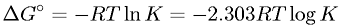Equations > Chemistry > Thermochemistry > Gibb's Free Energy Change in Terms of Gas Constant, Absolute Temperature, and Equilibrium Constant

Gibb's Free Energy Change in Terms of Gas Constant, Absolute Temperature, and Equilibrium ConstantLatex Code:

MathML Code:

 $\Delta {G}^{\circ }=-\mathrm{RT}\mathrm{ln}K=-2.303\mathrm{RT}\mathrm{log}K$

MathType 5.0: